# Nuclear Chemistry, Atoms: The Building Blocks of MatterDonna Chou
question

Transmutation

The change in the identity of an isotope due to a change in the number of its protons
question

Alpha Decay definition

When an isotope loses a particle with a 2 proton and a mass of 4
question

Beta Decay definition

When an isotope gains a proton through the loss of an electric charge on one of its neutrons
question

Gamma Decay definition

When an isotope decays to release only energy, not a particle
question

Positron

a positive particle with the same mass as an electron given off as a result of a proton changing to a neutron
question

Half-life

the amount of time it takes for half of a substance to decay into another substance
question

Electron

a negative sub atomic particle
question

Fission

the splitting of one large nucleus to create two smaller nuclei (two new elements with some mass being converted into energy)
question

Fusion

the combining of two smaller nuclei to create a larger nucleus (some mass is converted to energy)
question

Carbon Dating

the process using Carbon-14 to date materials that were once alive
question

Ion

a charged atom
question

isotope

an atom with fewer or more neutrons than the average form of that element
question

Alpha decay example

question

Beta decay example

question

Gamma Decay example

question

Atom

“indivisible” (Greek meaning), the smallest particle of an element that retains the chemical properties of that element
question

Dalton’s Atomic Postulate 1

All matter is made of atoms and atoms are indivisible, cannot be subdivided, created or destroyed
question

Dalton’s Atomic Postulate 2

All atoms of the same elements are identical in mass and properties (the same)
question

Dalton’s Atomic Postulate 3

Compounds are combinations in whole number ratios of two or more types of atoms
question

Dalton’s Atomic Postulate 4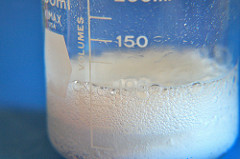In chemical reactions, atoms are combined, separated or rearranged
question

Dalton’s Atomic Postulate 5

Compounds are made in definite proportions
question

Law of Conservation of Mass

(Law of Lavoisier) in chemical reactions, the total mass is conserved
question

Law of Definite Proportion

(Proust) when a chemical compound is formed, their is a definite proportion of the atoms forming the compound (example: in table salt; sodium chloride; it ALWAYS has by mass, the same amount of sodium (Na , 39.34%) and chlorine (Cl, 60.66%)
question

Law of Multiple Proportions

if 2 or more different compounds are composed of the same 2 elements, then the ratio of the masses of the second element combined with a certain mass of the first element is always a ration of small whole numbers. (carbon and oxygen form carbon dioxide and carbon monoxide; the ratio of carbon to oxygen between dioxide to monoxide is always 2:1)
question

Atomic Number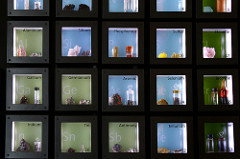number of protons of each atom of that element
question

Isotope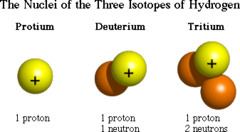atoms of the same element that have different numbers of neutrons in the nucleus (so different atomic masses) Tin has the most isotopes (10); although they differ in masses isotopes do not differ significantly in their chemical behavior
question

Mass number

the total number of protons plus neutrons that make up nucleus of an isotope
question

Nuclide

general term for a specific isotope of an element
question

Atomic Mass Unit

(amu) a unit of mass that describes the mass of an atom or molecule
question

Mole

(mol) the SI unit for amount of substance: the amount of a substance that contains as many particles as there are atoms in exactly 12g of carbon-12
question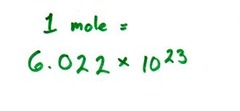the number of particles in exactly one mole of a pure substance (6.022 1415 x 10^23 or rounded to 6.022 x 10^23 = exactly what 12g of carbon-12 atoms contains)
question

Molar mass

equal to the atomic mass of the element; the mass of one mole of a pure substance is called the molar mass of that substance; written in units of g/mol; FOUND ON THE PERIODIC TABLE FOR EACH ELEMENT
question

Subatomic particles

what make up an atom; the three main are protons,neutrons, and electrons
question

Chemical Reaction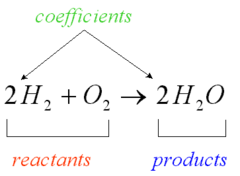transformation of a substance or substances into one or more new substances
question

Nucleus

a very small region located at the center of an atom
question

Proton

positively charged (+) particle equal in magnitude to the negative charge of an electron; at least one in every atom
question

Neutron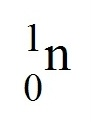neutral particle; at least one or two in every atom
question

Electron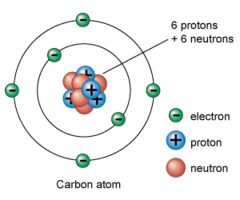negative charged particle (-); surrounds the nucleus in an occupied region
question

Cathode-ray tubes

glass tubes; in late 1800s, experiments were performed where electric current was passed through various gases at low temp; the resulting glowing stream was called cathode rays
question

Average Atomic Mass

weighted average of the atomic masses of the naturally occurring isotopes of an element
question

JJ Thomson

(1897) discovered electrons, charge to mass ratio and that all cathode rays are composed of identical negatively charged particles named electrons
question

Rutherford

(1911) discovered the nucleus, protons; Gold foil experiment
question

(1934) discovered the neutrons
question

Nuclear forces

the short-range proton-neutron, proton-proton, and neutron-neutron forces hold the nuclear particles together
question

Neutron equation (find # of neutrons)

Mass # – atomic # = # neutrons (protons+neutrons)-protons=neutrons
question

cathode rays

streams of negatively charged particles (electrons).
question

plum pudding model (Thomson)

JJ Thomson; negative electrons are spread evenly through the positive charge of the rest of the atom (think watermelon)
question

Robert A. Millikan (1909)

1. since atoms are electronically neutral they must contain a positive charge to balance the negative electrons 2. because electrons have so much less mass than atoms, atoms must contain other particles that account for most of their mass
question

1911 – Rutherford, Geiger and Marsden

bombarded a thin piece of gold foil with a narrow beam of alpha particle, some particles were deflected back to source – reasoned this was the nucleus and it was a very small part of atom because the rest of the particles passed through undisturbed
question

Nuclei

different elements differ in number of protons (positive charge) so the number of protons determines the atoms identity
question

nuclear forces

the short range proton-neutron; proton-proton and neutron-neutron forces that hold the nuclear particles together
question

picometer (pm)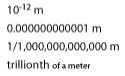Radius of an atom from the center of the nucleus to the outer portion of the electron cloud ; 1 pm = 10^-12(ten to the negative 12th power) meters or 10^-10 centimeters
question

atomic numbernumber of protons in each atom of that element
question

Designating isotopes

Identified by specifying their mass number in 2 ways: 1. Hyphen notation: hydrogen – 3 2. Nuclear symbol: 3 H 1 with mass number (neutron + protons) on top and atomic number (protons) on bottom
question

atomic mass units (amu)

all atoms compared to carbon-12 atoms (12 amu) so 1 amu = 1/12 of carbon
question

calculating average atomic mass

multiply mass of each type by the decimal fraction representing its % in the mixture 25% = .25 each weighing 2g; 75%=.75 each weighing 3g so (2g*.25)+(3gx.75)=2.75g
question

gram/mole conversions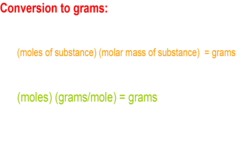(moles of substance) x molar mass = grams and (moles)(gram/moles)=grams
question

Rutherford model1911 model of the atom. small nucleus surrounded by electrons in orbit around it
question

Bohr atomic model

Suggest that electrons move in a definite path around the nucleus. 1913
question

Quantum mechanical model (electron cloud model)

a mathematical model and most accurate model of an atom used today
question

Dalton model

The first major model of the atom, developed in 1800.
question

Greek model

Basically, this model was a philosophical idea that everything could be broken down into one fundamental unit — the atom. It could not be tested at that point in time because the Greeks did not posses the technology to observe anything that was so microscopic such as the atom.
question

nucleons

the protons and neutrons in atomic nuclei
question

nuclide

the reference to an atom in nuclear chemistry; identified by the number of protons and neutrons in its nucleus
question

mass defect

difference between the mass of an atom and the sum of the masses of its protons, neutrons and electrons
question

what causes mass defect?

conversion of mass to energy upon formation of the nucleus
question

nuclear binding energythe energy release when a nucleus is formed from nucleons; E=mc^2
question

binding energy per nucleon

is the binding energy per of the nucleus divided by the number of nucleons it contains – the higher the binding energy the more tightly the nucleons are held together and are therefore more stable
question

band of stability

stable nuclei cluster over a range of neutron-proton ratios (the graph of elements and their isotopes plotted)
question

nuclear shell model

nucleons exist in different energy levels or shells in the nucleus
question

magic numbers

Numbers of nucleons that represent completed nuclear energy levels: 2, 8, 20, 28, 50, 82, and 126 – the most stable nuclides
question

nuclear reaction

reaction that affects the nucleus of an atom
question

transmutation

change in the identity of a nucleus as a result of a change in the number of its protons
question

the spontaneous disintegration of a nucleus into a slightly lighter nucleus, accompanied by emission of particles, electromagnetic radiation or both
question

Henri Becquerel

1896 wrapped a photographic plate in lightproof covering and place uranium on top of it. Figured out didn’t need sunlight to expose the plate, it was the radioactive decay of uranium that exposed the plate
question

question

an unstable nucleus that undergoes radioactive decay – all nuclides beyond atomic #83 are unstable and radioactive
question

alpha particle

two protons and two neutrons bound together and emitted from the nucleus during some kinds of radioactive decay; charge is 2+, represented by symbol
question

beta particle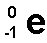an electron emitted from the nucleus during some kinds of ratioactive decay. atomic number 1+, mass stays the same
question

positron

particle that has the same mass as an electron but has a postive charege, and is emitted from the nucleus during some kinds of ratio active decay
question

electron capture

an inner orbital electron is captured by the nucleus of its own atom. the inner orbital electron combines with a proton and a neutron is formed; atomic number decreases by one but mass number doesn’t change (stays the same)
question

gamma rays

high energy electromagnetic waves emitted from a nucleus as it changes from an excited state to a ground energy state
question

penetration of particles

question

half life

time required for half of the atoms of a radioactive nuclide to decay
question

decay series

a series of ratioactive nuclides produced by successive radioactive decay until a stable nuclide is reached
question

parent nuclide

heaviest nuclide of each decay series
question

daughter nuclides

nuclides produced by the decay of the parent nuclides
question

question

artificial transmutations

bombardment of nuclei with charged and uncharged particles to make artificial radioactive nuclides; radioactive isotopes of all the natural elements have been produced this way
question

transuranium elements

elements with more than 92 protons in their nuclei – all are radioactive
question

roentgen (R)

unit used to measure nuclear radiation exposure
question

rem

unit used to measure the dose of any type of ionizing radiation that factors in the effect that the radiation has on human tissue
question

use exposure of film to measure the approximate radiation exposure of people working with radiation
question

Geiger-Muller counters

are instruments that detect radiation by counting electric pulses carried by gas ionized by radiation; typically used to detect beta particles, x rays and gamma radiation
question

scintillation counters

instruments that convert scintillating light to an electrical signal for detecting radiation
question

process by which the approximate age of an object is determined based on the amount of certain radioactive nuclides present: Carbon 14 is radioactive has half life of approximately 5715 years can be used to estimate the age of organic material to about 50,000 years
question

radioactive atoms that are incorporated into substances so that movement of the substances can be followed by radiation detectors – used to diagnose cancer etc
question

nuclear fission

nucleus of very heavy atom (uranium) split into 2 or more lighter nuclei; releases high amounts of energy
question

chain reaction

reaction in which the material that starts the reaction is also one of the products and can start another reaction
question

critical mass

minimum amount of nuclide that provides the number of neutrons needed to sustain a chain reaction
question

nuclear reactor

use controlled fission chain reactions to produce energy and radioactive nuclides
question

nuclear power plants

use energy as heat from nuclear reactors to produce electrical energy
question

shielding

radiation absorbing material that is used to decrease exposure to radiation especially gamma rays from nuclear reactors
question

control rods

neutron absorbing rods that help control the reaction by limiting the number of free neutrons
question

moderator